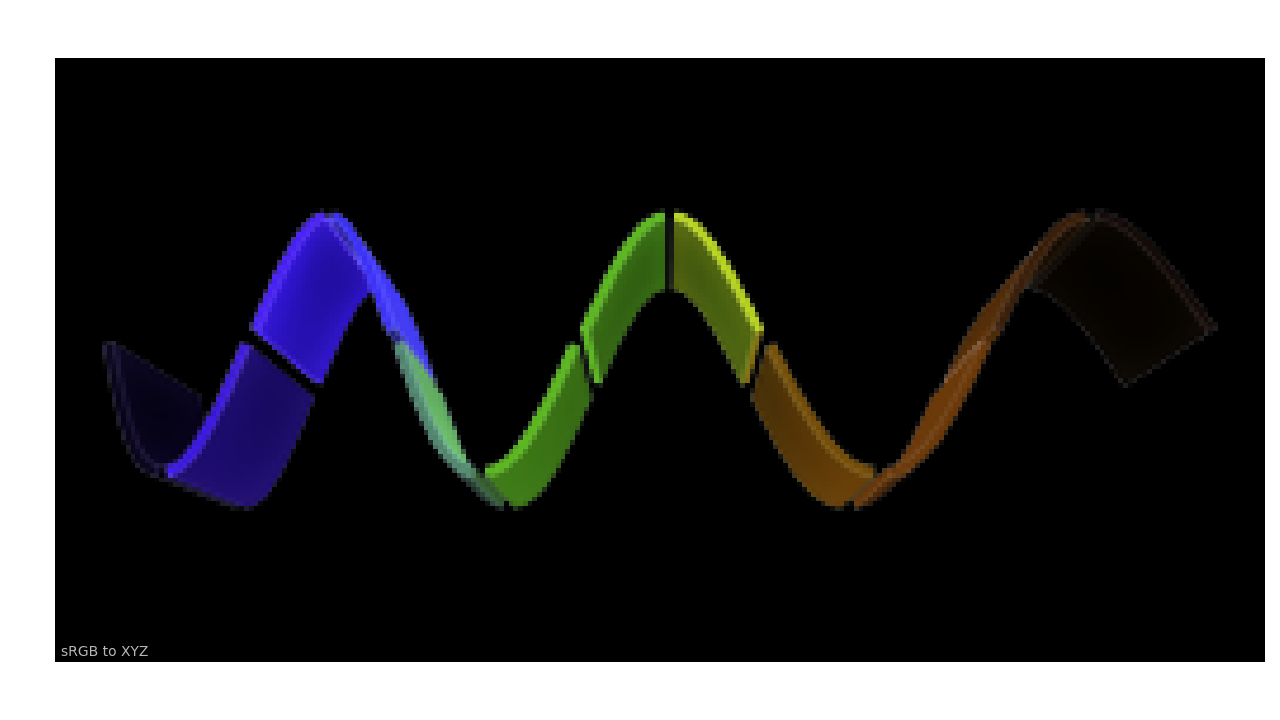# Basics¶

## N-Dimensional Arrays Support¶

Most of Colour definitions are fully vectorised and support n-dimensional arrays by leveraging Numpy.

While it is recommended to use ndarrays as input for the API objects, it is possible to use tuples or lists:

import colour

xyY = (0.4316, 0.3777, 0.1008)
colour.xyY_to_XYZ(xyY)

array([ 0.11518475,  0.1008    ,  0.05089373])

xyY = [0.4316, 0.3777, 0.1008]
colour.xyY_to_XYZ(xyY)

array([ 0.11518475,  0.1008    ,  0.05089373])

xyY = [
(0.4316, 0.3777, 0.1008),
(0.4316, 0.3777, 0.1008),
(0.4316, 0.3777, 0.1008),
]
colour.xyY_to_XYZ(xyY)

array([[ 0.11518475,  0.1008    ,  0.05089373],
[ 0.11518475,  0.1008    ,  0.05089373],
[ 0.11518475,  0.1008    ,  0.05089373]])


As shown in the above example, there is widespread support for n-dimensional arrays:

import numpy as np

xyY = np.array([0.4316, 0.3777, 0.1008])
xyY = np.tile(xyY, (6, 1))
colour.xyY_to_XYZ(xyY)

array([[ 0.11518475,  0.1008    ,  0.05089373],
[ 0.11518475,  0.1008    ,  0.05089373],
[ 0.11518475,  0.1008    ,  0.05089373],
[ 0.11518475,  0.1008    ,  0.05089373],
[ 0.11518475,  0.1008    ,  0.05089373],
[ 0.11518475,  0.1008    ,  0.05089373]])

colour.xyY_to_XYZ(xyY.reshape((2, 3, 3)))

array([[[ 0.11518475,  0.1008    ,  0.05089373],
[ 0.11518475,  0.1008    ,  0.05089373],
[ 0.11518475,  0.1008    ,  0.05089373]],

[[ 0.11518475,  0.1008    ,  0.05089373],
[ 0.11518475,  0.1008    ,  0.05089373],
[ 0.11518475,  0.1008    ,  0.05089373]]])


Which enables image processing:

import colour.plotting

RGB = RGB[..., 0:3]  # Discarding alpha channel.
XYZ = colour.sRGB_to_XYZ(RGB)
colour.plotting.plot_image(XYZ, text_parameters={'text': 'sRGB to XYZ'})## Domain-Range Scales¶

Note

This section has important information.

Colour adopts 4 main input domains and output ranges:

• Scalars usually in domain-range [0, 1] (or [0, 10] for Munsell Value).
• Percentages usually in domain-range [0, 100].
• Degrees usually in domain-range [0, 360].
• Integers usually in domain-range [0, 2**n -1] where n is the bit depth.

It is error prone but it is also a direct consequence of the inconsistency of the colour science field itself. We have discussed at length about this and we were leaning toward normalisation of the whole API to domain-range [0, 1], we never committed for reasons highlighted by the following points:

• Colour Scientist performing computations related to Munsell Renotation System would be very surprised if the output Munsell Value was in range [0, 1] or [0, 100].
• A Visual Effect Industry artist would be astonished to find out that conversion from CIE XYZ to sRGB was yielding values in range [0, 100].

However benefits of having a consistent and predictable domain-range scale are numerous thus with Colour 0.3.12 we have introduced a mechanism to allow users to work within one of the two available domain-range scales.

### Scale - Reference¶

‘Reference’ is the default domain-range scale of Colour, objects adopt the implemented reference, i.e. paper, publication, etc.., domain-range scale.

The ‘Reference’ domain-range scale is inconsistent, e.g. colour appearance models, spectral conversions are typically in domain-range [0, 100] while RGB models will operate in domain-range [0, 1]. Some objects, e.g. colour.colorimetry.lightness_Fairchild2011() definition have mismatched domain-range: input domain [0, 1] and output range [0, 100].

### Scale - 1¶

‘1’ is a domain-range scale converting all the relevant objects from Colour public API to domain-range [0, 1]:

• Scalars in domain-range [0, 10], e.g Munsell Value are scaled by 10.
• Percentages in domain-range [0, 100] are scaled by 100.
• Degrees in domain-range [0, 360] are scaled by 100.
• Integers in domain-range [0, 2**n -1] where n is the bit depth are scaled by 2**n -1.

Warning

The conversion to ‘1’ domain-range scale is a soft normalisation and similarly to the ‘Reference’ domain-range scale it is normal that you encounter values exceeding 1, e.g. High Dynamic Range Imagery (HDRI) or negative values, e.g. out-of-gamut RGB colourspace values.

### Understanding the Domain-Range Scale of an Object¶

Using colour.adaptation.chromatic_adaptation_CIE1994() definition docstring as an example, the Notes section features two tables.

The first table is for the domain, and lists the input arguments affected by the two domain-range scales and which normalisation they should adopt depending the domain-range scale in use:

Domain Scale - Reference Scale - 1
XYZ_1 [0, 100] [0, 1]
Y_o [0, 100] [0, 1]

The second table is for the range and lists the return value of the definition:

Range Scale - Reference Scale - 1
XYZ_2 [0, 100] [0, 1]

### Working with the Domain-Range Scales¶

The current domain-range scale is returned with the colour.get_domain_range_scale() definition:

import colour

colour.get_domain_range_scale()

u'reference'


Changing from the ‘Reference’ default domain-range scale to ‘1’ is done with the colour.set_domain_range_scale() definition:

XYZ_1 = [28.00, 21.26, 5.27]
xy_o1 = [0.4476, 0.4074]
xy_o2 = [0.3127, 0.3290]
Y_o = 20
E_o1 = 1000
E_o2 = 1000

array([ 24.03379521,  21.15621214,  17.64301199])

colour.set_domain_range_scale('1')

XYZ_1 = [0.2800, 0.2126, 0.0527]
Y_o = 0.2

array([ 0.24033795,  0.21156212,  0.17643012])


The output tristimulus values with the ‘1’ domain-range scale are equal to those from ‘Reference’ default domain-range scale divided by 100.

Passing incorrectly scaled values to the colour.adaptation.chromatic_adaptation_CIE1994() definition would result in unexpected values and a warning in that case:

colour.set_domain_range_scale('Reference')


File "<ipython-input-...>", line 4, in <module>
E_o2)
warning(('"Y_o" luminance factor must be in [18, 100] domain, '
/colour-science/colour/colour/utilities/verbose.py:207: ColourWarning: "Y_o" luminance factor must be in [18, 100] domain, unpredictable results may occur!
warn(*args, **kwargs)
array([ 0.17171825,  0.13731098,  0.09972054])


Setting the ‘1’ domain-range scale has the following effect on the colour.adaptation.chromatic_adaptation_CIE1994() definition:

As it expects values in domain [0, 100], scaling occurs and the relevant input values, i.e. the values listed in the domain table, XYZ_1 and Y_o are converted from domain [0, 1] to domain [0, 100] by colour.utilities.to_domain_100() definition and conversely return value XYZ_2 is converted from range [0, 100] to range [0, 1] by colour.utilities.from_range_100() definition.

A convenient alternative to the colour.set_domain_range_scale() definition is the colour.domain_range_scale context manager and decorator. It temporarily overrides Colour domain-range scale with given scale value:

with colour.domain_range_scale('1'):

[ 0.24033795  0.21156212  0.17643012]